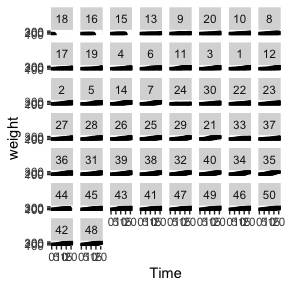# Intro

The puspose of this note is to make a connection between R functions and the mathematical notation of linear and (non)linear mixed models. One goal is to produce a table that maps R functions with notation for these models.

This document is a work in progress.

## Linear Model

A linear model can be expressed as

$y = X \beta + \epsilon$

Typically, we assume that the errors are normally distributed, independent and they have constant variance.

$\epsilon \sim N(0, \sigma^2)$

Note: The covariance of the errors $$Cov(\mathbf{\epsilon})$$ where $$\mathbf{\epsilon}$$ is an $$n \times 1$$ vector is $$\sigma^2 \mathbf{I}_n$$.

In R we can fit the following model

x <- rnorm(20)
y <- 1 + 2 * x + rnorm(20, sd = 0.5)
plot(x, y)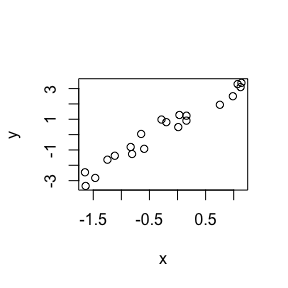fit <- lm(y ~ x)

In order to extract the estimate for $$\beta$$, this is $$\hat{\beta}$$, we can use the function coef. In order to extract the estiamte for $$\sigma$$ (again, strictly $$\hat{\sigma}$$), we can use the function sigma. In order to extract the covariance of $$\hat{\beta}$$, this is $$Cov(\hat{\beta})$$, we can use the function vcov.

The covariance for $$\hat{\beta}$$ is defined as

$Cov(\hat{\beta}) = \hat{\sigma}^2 (\mathbf{X'X})^{-1}$

Obtaining this matrix is important for simulation as it provides information on the variances for the parameters in the model as well as the covariances.

## Print beta hat
coef(fit)
## (Intercept)           x
##   0.8059534   2.1388860
## Print sigma
sigma(fit)
##  0.4212005
## Print the covariance matrix of beta hat
vcov(fit)
##             (Intercept)           x
## (Intercept) 0.009551574 0.002697391
## x           0.002697391 0.010682887

There are other functions in R which are also useful. The function fitted extracts the fitted values or $$\hat{y} = \mathbf{X}\hat{\beta}$$, model.matrix extracts $$\mathbf{X}$$ and residuals extracts $$\hat{e}$$ (or resid).

In addition, in the nlraa package I have the function ‘var_cov’ which extracts the complete variance of the residuals. In this case it is a simple diagonal matrix multiplied by $$\hat{\sigma}^2$$.

## Extract the variance covariance of the residuals (which is also of the response)
lm.vc <- var_cov(fit)
## Print just the first 5 observations
round(lm.vc[1:5,1:5],2)
##      [,1] [,2] [,3] [,4] [,5]
## [1,] 0.18 0.00 0.00 0.00 0.00
## [2,] 0.00 0.18 0.00 0.00 0.00
## [3,] 0.00 0.00 0.18 0.00 0.00
## [4,] 0.00 0.00 0.00 0.18 0.00
## [5,] 0.00 0.00 0.00 0.00 0.18
## Visualize the matrix. This is a 20 x 20 matrix.
## It is easier to visualize in the log-scale
## Note: log(0) becomes -Inf, but not shown here
image(log(lm.vc[,ncol(lm.vc):1]))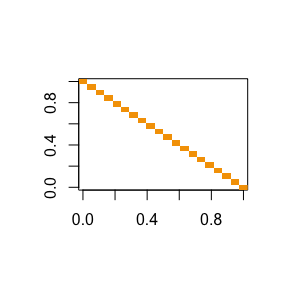In the previous image, each orange square represents the estimate of the residual variance ($$\sigma^2$$) and there are 20 squares because there are 20 observations.

It might seem silly for a model such as this one to extract a matrix which is simlpy a diagonal identity matrix multiplied by the scalar $$\hat{\sigma}^2$$, but this will become more clear as we move into more complex models.

So far,

r.function beta cov.beta sigma X y.hat e cov.e
lm coef vcov sigma model.matrix fitted residuals var_cov

## Generalized least squares (gls)

Note: The models that can be fitted using gls should not be confused with the ones fitted using glm, which are generalized linear models.

The function gls in the nlme package fits linear models, but in which the covariance matrix of the residuals (also called errors) is more flexible.

The model is still

$y = X \beta + \epsilon$

But now the errors have a more flexible covariance structure.

$\epsilon \sim N(0, \Sigma)$

How do we extract $$\Sigma$$ from a fitted model?

We will again use the function var_cov (there is a function in nlme called getVarCov, but there are many cases which it does not handle).

For the next example I will use the ChickWeight dataset.

## ChickWeight example
data(ChickWeight)
ggplot(data = ChickWeight, aes(Time, weight)) + geom_point()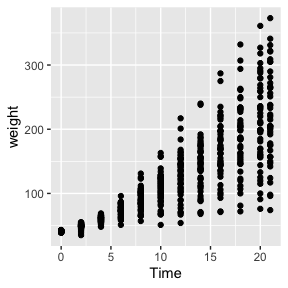Clearly, the variance increases as the weight increases and it would be a good approach to consider this in the modeling process. The code below fits the variance of the residuals (or the response) as a function of the fitted values. We could choose and evaluate different variance functions and determine which one is better, but for the purpose of illustrating the connection between R functions and mathematical notation this is enough.

## One possible model is
fit.gls <- gls(weight ~ Time, data = ChickWeight,
weights = varPower())
fit.gls.vc <- var_cov(fit.gls)
## Note: the function getVarCov fails for this object
## Visualize the first few observations
fit.gls.vc[1:10,1:10]
##           [,1]     [,2]     [,3]     [,4]     [,5]     [,6]     [,7]     [,8]
##  [1,] 3.530572  0.00000  0.00000   0.0000   0.0000   0.0000    0.000    0.000
##  [2,] 0.000000 15.12026  0.00000   0.0000   0.0000   0.0000    0.000    0.000
##  [3,] 0.000000  0.00000 47.53714   0.0000   0.0000   0.0000    0.000    0.000
##  [4,] 0.000000  0.00000  0.00000 122.3036   0.0000   0.0000    0.000    0.000
##  [5,] 0.000000  0.00000  0.00000   0.0000 273.3773   0.0000    0.000    0.000
##  [6,] 0.000000  0.00000  0.00000   0.0000   0.0000 550.6203    0.000    0.000
##  [7,] 0.000000  0.00000  0.00000   0.0000   0.0000   0.0000 1023.508    0.000
##  [8,] 0.000000  0.00000  0.00000   0.0000   0.0000   0.0000    0.000 1785.058
##  [9,] 0.000000  0.00000  0.00000   0.0000   0.0000   0.0000    0.000    0.000
## [10,] 0.000000  0.00000  0.00000   0.0000   0.0000   0.0000    0.000    0.000
##           [,9]    [,10]
##  [1,]    0.000    0.000
##  [2,]    0.000    0.000
##  [3,]    0.000    0.000
##  [4,]    0.000    0.000
##  [5,]    0.000    0.000
##  [6,]    0.000    0.000
##  [7,]    0.000    0.000
##  [8,]    0.000    0.000
##  [9,] 2955.968    0.000
## [10,]    0.000 4688.938
## Store for visualization
## only the first 12 observations
vc2 <- fit.gls.vc[1:12,1:12]
round(vc2,0)
##       [,1] [,2] [,3] [,4] [,5] [,6] [,7] [,8] [,9] [,10] [,11] [,12]
##  [1,]    4    0    0    0    0    0    0    0    0     0     0     0
##  [2,]    0   15    0    0    0    0    0    0    0     0     0     0
##  [3,]    0    0   48    0    0    0    0    0    0     0     0     0
##  [4,]    0    0    0  122    0    0    0    0    0     0     0     0
##  [5,]    0    0    0    0  273    0    0    0    0     0     0     0
##  [6,]    0    0    0    0    0  551    0    0    0     0     0     0
##  [7,]    0    0    0    0    0    0 1024    0    0     0     0     0
##  [8,]    0    0    0    0    0    0    0 1785    0     0     0     0
##  [9,]    0    0    0    0    0    0    0    0 2956     0     0     0
## [10,]    0    0    0    0    0    0    0    0    0  4689     0     0
## [11,]    0    0    0    0    0    0    0    0    0     0  7173     0
## [12,]    0    0    0    0    0    0    0    0    0     0     0  8767
## The variance increases as weight increases
## Visualize the variance-covariance of the errors
## This is only for the first 12 observations
## It is easier to visualize in the log scale
## Note: log(0) becomes -Inf, but not shown here
image(log(vc2[,ncol(vc2):1]),
main = "First 12 observations, Cov(resid)")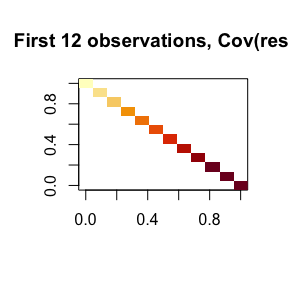## For all observations
image(log(fit.gls.vc[,ncol(fit.gls.vc):1]),
main = "All observations, Cov(resid)")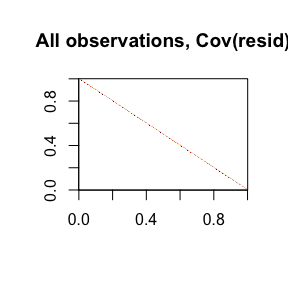Since not only the variance is increasing, but also the data comes from different chick and is thus correlated, we could fit the following model.

## Adding the correlation
fit.gls2 <- gls(weight ~ Time, data = ChickWeight,
weights = varPower(),
correlation = corCAR1(form = ~ Time | Chick))
## Extract the variance-covariance of the residuals
## Note: getVarCov fails for this object
fit.gls2.vc <- var_cov(fit.gls2)
## Visualize the first few observations
round(fit.gls2.vc[1:13,1:13],0)
##       [,1] [,2] [,3] [,4] [,5] [,6] [,7] [,8] [,9] [,10] [,11] [,12] [,13]
##  [1,]   89  129  178  236  302  377  462  555  658   770   890   954     0
##  [2,]  129  194  267  353  452  565  692  832  985  1152  1333  1428     0
##  [3,]  178  267  379  501  642  803  982 1181 1400  1637  1893  2029     0
##  [4,]  236  353  501  684  877 1096 1341 1613 1911  2235  2585  2769     0
##  [5,]  302  452  642  877 1160 1449 1774 2133 2527  2956  3418  3663     0
##  [6,]  377  565  803 1096 1449 1869 2287 2750 3259  3811  4408  4723     0
##  [7,]  462  692  982 1341 1774 2287 2888 3473 4115  4813  5566  5964     0
##  [8,]  555  832 1181 1613 2133 2750 3473 4310 5106  5972  6907  7400     0
##  [9,]  658  985 1400 1911 2527 3259 4115 5106 6241  7300  8443  9046     0
## [10,]  770 1152 1637 2235 2956 3811 4813 5972 7300  8809 10188 10916     0
## [11,]  890 1333 1893 2585 3418 4408 5566 6907 8443 10188 12158 13026     0
## [12,]  954 1428 2029 2769 3663 4723 5964 7400 9046 10916 13026 14177     0
## [13,]    0    0    0    0    0    0    0    0    0     0     0     0    89
## Visualize the variance-covariance of the errors
## On the log-scale
## Reorder and select
vc2.36 <- fit.gls2.vc[1:36,1:36]
image(log(vc2.36[,ncol(vc2.36):1]),
main = "Covariance matrix of residuals \n for the first three Chicks (log-scale)")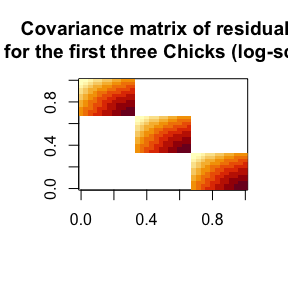Let’s plot the data, by Chick. this shows that there is a high temporal correlation as we would expect. Chicks which have a higher weight (than others) at given time, tend to still be higher at a later time.

ggplot(data = ChickWeight, aes(x = Time, y = weight)) +
facet_wrap( ~ Chick) +
geom_point()